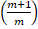# A convex lens of focal length f produces a real image of size m times the size of the object. Then the object distance is (a)f (b) (m+1)f (c)(d)## Question ID - 153988 :- A convex lens of focal length f produces a real image of size m times the size of the object. Then the object distance is (a)f (b) (m+1)f (c)(d)3537f

Next Question :
 The absolute refractive index of a medium is 1.5. Then what would be the velocity of light in this medium ? (a) 2 x 108 m/s (b) 1.5 x 108 m/s (c) 3.5 x 108 m/s (d) 2.5 x 108 m/s